# Galvanic Cells or Voltaic Cells Chemistry Tutorial

## Key Concepts

• Galvanic Cell (or Voltaic Cell) is a type of electrochemical cell1 that converts chemical energy of oxidants and reductants into electrical energy
• The redox reaction must be spontaneous in order to produce electricity:
For a standard galvanic cell (voltaic cell):

E0 for galvanic cell is positive

that is, E0(cell) > 0

• E0(cell), is referred to as the standard cell's potential difference or the cell's electromotive force (emf), and is the maximum voltage that can be produced by the redox cell.
In practice, the cell's emf depends on temperature and the concentration of reactants and products.

(i) If the concentration of reactants increases relative to the concentration of products, the cell reaction becomes more spontaneous and the cell's emf increases.

(ii) As the cell operates, the reactants are used up as more product is formed causing the cell's emf to decrease.

• A typical galvanic cell (voltaic cell) consists of:

(i) Electrolytes: conducting substances containing the species that will take part in the oxidation and reduction reactions.

(ii) Two Electrodes2: conductors used to permit the flow of electrons in an electrochemical cell and provide surfaces at which the oxidation and reduction reactions can take place.

(a) One electrode is negative (-) and is called the anode.
Oxidation occurs at the anode.

(b) The other electrode is positive (+) and is called the cathode.
Reduction occurs at the cathode.

(iii) A conducting wire, or lead, connects the two electrodes allowing electrons to flow from the anode to the cathode.

Connecting these wires to a voltmeter will measure the voltage produced by the galvanic cell (voltaic cell).

(iv) Salt bridge: allows for migration of ions to complete the electrical circuit

The salt used in the salt bridge must3 :

(a) be soluble in each half-cell's solvent

For example, salt must be soluble in water if aqueous solutions are used in the half-cell.

(b) not react with any species in either half-cell.

For these reasons, salts of Group 1 (alkali metals) are commonly used in the salt bridge because the salts of all Group 1 metals are soluble in water, and, Group 1 metal ions are very weak oxidants so will not react with species in solution.
Similarly, nitrate ions, NO3-, are often used in the salt bridge because all nitrates are soluble in water.

Potassium nitrate, KNO3, is often used in the salt bridge in school laboratories.

No ads = no money for us = no free stuff for you!

## Typical Galvanic Cell or Voltaic Cell

Consider a galvanic cell (voltaic cell) made up of two half-cells as shown in the diagram below: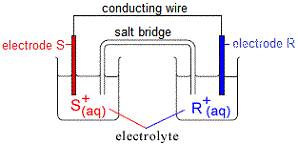One half-cell contains an electrode made of metal S partly submerged in an electrolyte containing its ions, S+(aq).

The other half-cell contains an electrode made of metal R partly submerged in an electrolyte containing its ions, R+(aq).

If metal R is easier to oxidise than metal S (metal R is the better reductant, or a better reducing agent) then :

(i) metal R in one half-cell will be oxidised (lose electrons)

R(s) → R+(aq) + e-

(ii) metal ions in the other half-cell, S+, will be reduced (gain electrons)

S+(aq) + e- → S(s)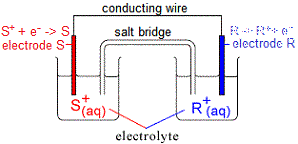The electrons produced by the oxidation of metal R flow through the conducting wire connecting electrode R to electrode S.

At electrode S, these electrons are used to reduce S+(aq) in the electrolyte.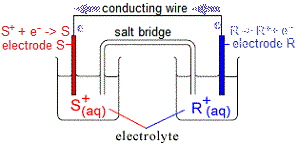However, electrons can only flow IF the circuit is complete, that is, if a salt bridge for example is used to connect the electrolyte solution in one half-cell to the electrolyte solution in the other half cell.

If the salt bridge contains potassium chloride, KCl(aq), then:

(i) cations (K+) flow through the salt bridge towards the half-cell in which cations are being removed (that is, towards electrolyte S+)

(ii) anions (Cl-) flow through the salt bridge towards the half-cell in which electrons are being removed (that is, towards electrolyte R+)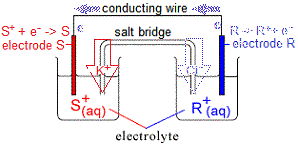The electrode at which the negatively charged electrons are being produced (electrode R) will be negative.
The other electrode, S, at which electrons are being used up will be positive.

For a galvanic cell (voltaic cell) which is producing electricity:

• Negative electrode is called the anode.
(i) Oxidation occurs at the anode.
(ii) Anions (negatively charged ions) migrate towards the anode.
• Positive electrode is called the cathode.
(i) Reduction occurs at the cathode.
(ii) Cations (positively charged ions) migrate towards the cathode.

A labelled diagram of this galvanic cell (or voltaic cell) is shown below: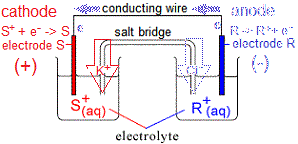If a voltmeter is placed in the circuit as shown in the diagram below: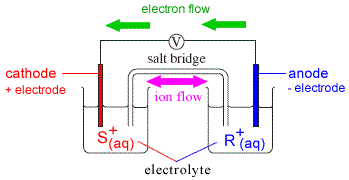then the voltage (electromotive force or emf) produced by this galvanic cell (voltaic cell) can be measured.

If the electrolyte in each half-cell is a standard solution (approximated as 1 mol L-1 at 25°C), then we can look up the value of the standard electrode potential for each half-cell and use these to calculate the voltage (emf) for the overall redox reaction occurring in the galvanic cell (voltaic cell):

 overall cell(redox) cathode(reduction) S+(aq) + e- → S(s) Eo(reduction) anode(oxidation) R(s) → e- + R+(aq) Eo(oxidation) S+(aq) + R(s) → S(s) + R+(aq) Eo(cell) > 0

Eo(cell) = Eo(reduction) + Eo(oxidation)

Note that in order for the galvanic cell to produce electricity, the reaction must be spontaneous, and the value of Eo must be positive (that is, greater than 0)

Do you know this?

Play the game now!

Do you understand this?

Take the test now!

## Worked Example : The Daniell Cell

In 1836 Professor John Daniell adopted a two-cell approach to produce electricity.
The Daniell Cell is divided into 2 half-cells connected by a wire and a salt bridge to complete the electrical circuit.

 Cathode Half-cell (+) electrode : copper metal, Cu(s) electrolyte : 1M CuSO4(aq) reduction reaction: Cu2+(aq) + 2e- → Cu(s)     Eo = +0.35 V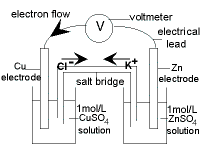salt bridge: KCl(s) KCl is soluble in the water. Neither K+(aq) nor Cl-(aq) will react with the other species present in each half-cell. Anode Half-cell (-) electrode : zinc metal, Zn(s) electrolyte : 1M ZnSO4(aq) oxidation reaction: Zn(s) → Zn2+(aq) + 2e-     E0 = +0.76 V

 Cathode half cell:(reduction) Cu2+(aq) + 2e- → Cu(s) Eo = +0.35 V Anode half-cell:(oxidation) Zn(s) → Zn2+(aq) + 2e- Eo = +0.76 V Daniell Cell:(redox) Cu2+(aq) + Zn(s) → Cu(s) + Zn2+(aq) Eocell = (+0.35) + (+0.76)       = +1.11 V

• At the negative electrode (anode), zinc is oxidised to zinc ions.
It is easier to oxidise zinc metal than it is to oxidise copper metal so the zinc metal is oxidised.
In other words, zinc (Zn(s)) is a stronger reductant (stronger reducing agent) than copper (Cu(s))
• The zinc anode disintegrates in time.
Over time, the zinc metal from the electrode is being converted to zinc ions, and these ions become part of the electrolyte solution.
Over time, the mass of the zinc electrode will decrease!
• At the positive electrode (cathode), copper ions are reduced to copper atoms.
Reduction occurs at the cathode.
At the cathode a species must gain electrons.
Copper metal, Cu(s) won't gain electrons, BUT, copper(II) ions in the electrolyte solution, Cu2+(aq), certainly can gain 2 electrons.
• Copper is deposited on the copper cathode in time.
Copper(II) ions, Cu2+(aq) continue to gain 2 electrons to form solid copper, Cu(s).
This reaction occurs on the copper electrode.
The "new" copper atoms stick to the "old" copper atoms of the electrode.
As the cell operates the mass of the copper electrode increases!
• Electrons flow from the zinc anode to the copper cathode.
Electrons are produced by the oxidation reaction occurring at the anode (zinc electrode).
Electrons flow through the conducting wire from the anode to the cathode.
At the cathode (copper electrode) these electrons are used to reduce copper(II) ions to copper atoms.
• In the overall redox reaction zinc is donating electrons to copper ions.
• This redox reaction occurs spontaneously, Eo is positive.
• This redox reaction is predicted to produce 1.11 V of electricity.

Can you apply this?

Join AUS-e-TUTE!

Take the exam now!

1. There are 2 types of electrochemical cells, the galvanic (voltaic) cells discussed here which convert chemical energy into electrical energy, and, electrolytic cells which convert electrical energy into chemical energy.

2. The are many different types of electrodes that can be used in galvanic (voltaic) cells.
These include:

• metal-metal ion electrodes (metal is in contact with its ions in solution)
• gas-ion electrodes (a gas in contact with its anion or cation in solution)
• metal-insoluble salt-anion electrodes (a metal in contact with one of its insoluble salts and also with a solution containing the anion of the salt)
• inert, "oxidation-reduction" electrodes (strip of inert conductor such as platinum in contact with a solution containing ions of a substance in two different oxidation states)
• membrane electrodes (a thin membrane, such as glass, separates the two electrolyte solutions)

3. There are other considerations regarding the salt chosen for the salt bridge, such as the rate of migration of the cations and ions, but these are probably outside the scope of a high school chemistry course.